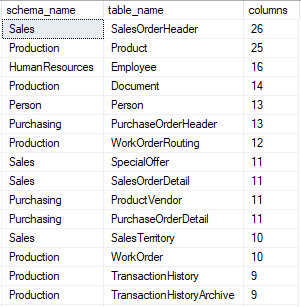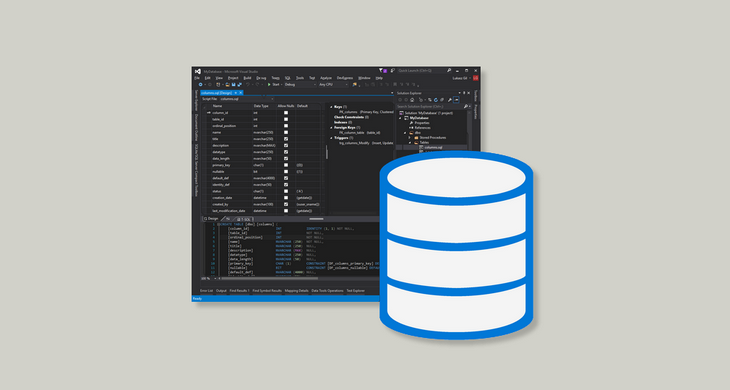## 6 Useful SQL Server Data Dictionary Queries Every DBA Should Have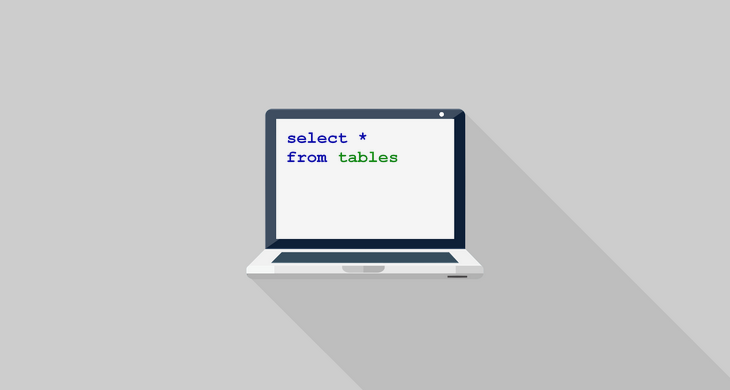This is a list of handy SQL queries to the SQL Server data dictionary. You can also find 100+ other useful queries here.

## 1. List of tables with number of rows and comments

This query returns list of tables in a database sorted by schema and table name with comments and number of rows in each table.

### Query

``````select schema_name(tab.schema_id) as schema_name,
tab.name as table_name,
tab.create_date as created,
tab.modify_date as last_modified,
p.rows as num_rows,
from sys.tables tab
inner join (select distinct
p.object_id,
sum(p.rows) rows
from sys.tables t
inner join sys.partitions p
on p.object_id = t.object_id
group by p.object_id,
p.index_id) p
on p.object_id = tab.object_id
left join sys.extended_properties ep
on tab.object_id = ep.major_id
and ep.name = 'MS_Description'
and ep.minor_id = 0
and ep.class_desc = 'OBJECT_OR_COLUMN'
order by schema_name,
table_name
``````

### Rows

One row represents one table. All tables will be included.

### Columns

Column Meaning
SCHEMA_NAME Schema name.
TABLE_NAME Table name.
CREATED Table creation date and time.
LAST_MODIFIED Table last modification date and time.
NUM_ROWS Number of rows in the table.

### Sample results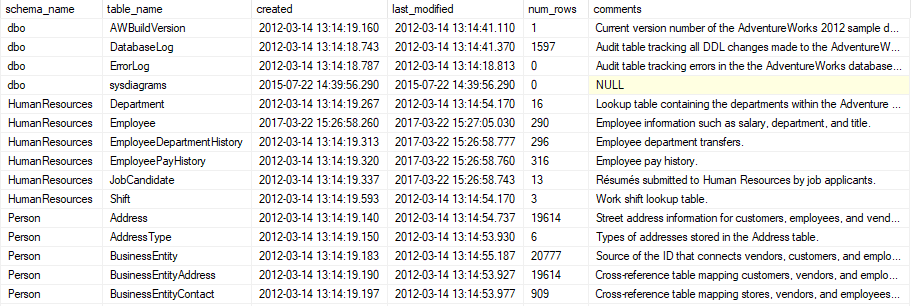## 2. List of views with definition and comments

This query returns list of database views with their definition SQL and a comment.

### Query

``````select schema_name(v.schema_id) as schema_name,
v.name as view_name,
v.create_date as created,
v.modify_date as last_modified,
m.definition,
from sys.views v
left join sys.extended_properties ep
on v.object_id = ep.major_id
and ep.name = 'MS_Description'
and ep.minor_id = 0
and ep.class_desc = 'OBJECT_OR_COLUMN'
inner join sys.sql_modules m
on m.object_id = v.object_id
order by schema_name,
view_name
``````

### Rows

One row represents one view.

### Columns

Column Meaning
SCHEMA_NAME Schema name.
VIEW_NAME View name.
CREATED View creation date and time.
LAST_MODIFIED View last modification date and time.
DEFINITION View definition (SQL query).

### Sample results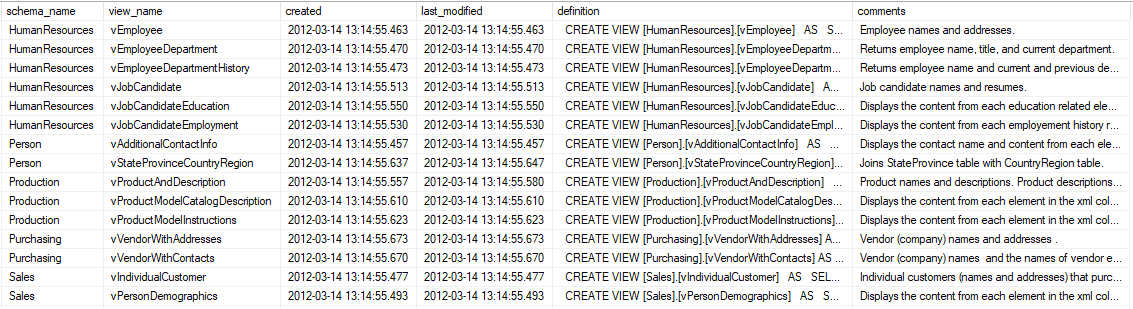## 3. Table columns details

This query returns list of tables and their columns with details.

### Query

``````select schema_name(tab.schema_id) as schema_name,
tab.name as table_name,
col.name as column_name,
t.name as data_type,
t.name +
case when t.is_user_defined = 0 then
isnull('(' +
case when t.name in ('binary', 'char', 'nchar',
'varchar', 'nvarchar', 'varbinary') then
case col.max_length
when -1 then 'MAX'
else
case when t.name in ('nchar',
'nvarchar') then
cast(col.max_length/2
as varchar(4))
else cast(col.max_length
as varchar(4))
end
end
when t.name in ('datetime2', 'datetimeoffset',
'time') then
cast(col.scale as varchar(4))
when t.name in ('decimal', 'numeric') then
cast(col.precision as varchar(4)) + ', ' +
cast(col.scale as varchar(4))
end + ')', '')
else ':' +
(select c_t.name +
isnull('(' +
case when c_t.name in ('binary', 'char',
'nchar', 'varchar', 'nvarchar',
'varbinary') then
case c.max_length
when -1 then 'MAX'
else
case when t.name in
('nchar',
'nvarchar') then
cast(c.max_length/2
as varchar(4))
else cast(c.max_length
as varchar(4))
end
end
when c_t.name in ('datetime2',
'datetimeoffset', 'time') then
cast(c.scale as varchar(4))
when c_t.name in ('decimal', 'numeric') then
cast(c.precision as varchar(4)) + ', '
+ cast(c.scale as varchar(4))
end + ')', '')
from sys.columns as c
inner join sys.types as c_t
on c.system_type_id = c_t.user_type_id
where c.object_id = col.object_id
and c.column_id = col.column_id
and c.user_type_id = col.user_type_id
)
end as data_type_ext,
case when col.is_nullable = 0 then 'N'
else 'Y' end as nullable,
case when def.definition is not null then def.definition
else '' end as default_value,
case when pk.column_id is not null then 'PK'
else '' end as primary_key,
case when fk.parent_column_id is not null then 'FK'
else '' end as foreign_key,
case when uk.column_id is not null then 'UK'
else '' end as unique_key,
case when ch.check_const is not null then ch.check_const
else '' end as check_contraint,
cc.definition as computed_column_definition,
from sys.tables as tab
left join sys.columns as col
on tab.object_id = col.object_id
left join sys.types as t
on col.user_type_id = t.user_type_id
left join sys.default_constraints as def
on def.object_id = col.default_object_id
left join (
select index_columns.object_id,
index_columns.column_id
from sys.index_columns
inner join sys.indexes
on index_columns.object_id = indexes.object_id
and index_columns.index_id = indexes.index_id
where indexes.is_primary_key = 1
) as pk
on col.object_id = pk.object_id
and col.column_id = pk.column_id
left join (
select fc.parent_column_id,
fc.parent_object_id
from sys.foreign_keys as f
inner join sys.foreign_key_columns as fc
on f.object_id = fc.constraint_object_id
group by fc.parent_column_id, fc.parent_object_id
) as fk
on fk.parent_object_id = col.object_id
and fk.parent_column_id = col.column_id
left join (
select c.parent_column_id,
c.parent_object_id,
'Check' check_const
from sys.check_constraints as c
group by c.parent_column_id,
c.parent_object_id
) as ch
on col.column_id = ch.parent_column_id
and col.object_id = ch.parent_object_id
left join (
select index_columns.object_id,
index_columns.column_id
from sys.index_columns
inner join sys.indexes
on indexes.index_id = index_columns.index_id
and indexes.object_id = index_columns.object_id
where indexes.is_unique_constraint = 1
group by index_columns.object_id,
index_columns.column_id
) as uk
on col.column_id = uk.column_id
and col.object_id = uk.object_id
left join sys.extended_properties as ep
on tab.object_id = ep.major_id
and col.column_id = ep.minor_id
and ep.name = 'MS_Description'
and ep.class_desc = 'OBJECT_OR_COLUMN'
left join sys.computed_columns as cc
on tab.object_id = cc.object_id
and col.column_id = cc.column_id
order by schema_name,
table_name,
column_name;
``````

### Rows

One row represents one table column.

### Columns

Column Meaning
SCHEMA_NAME Schema name.
TABLE_NAME Table name.
COLUMN_NAME Column name.
DATA_TYPE Data type. For instance, varchar or decimal.
DATA_TYPE_EXT Data type with information about scale/precision or string length. For instance, varchar(100) or decimal(8, 2).
NULLABLE Nullable flag. "Y" if column is nullable, "N" if column is not nullable.
DEFAULT_VALUE Column default value.
PRIMARY_KEY Primary key flag. "PK" when column is part of table primary key.
FOREIGN_KEY Foreign key flag. "FK" when column is part of foreign key.
UNIQUE_KEY Unique key flag. "UK" when column is part of unique key.
CHECK_CONSTRAINT Check constraint flag. "Check" when column is part of check constraint.
COMPUTED_COLUMN_DEFINITION Computed column definition (not null only if column is computed).

### Sample results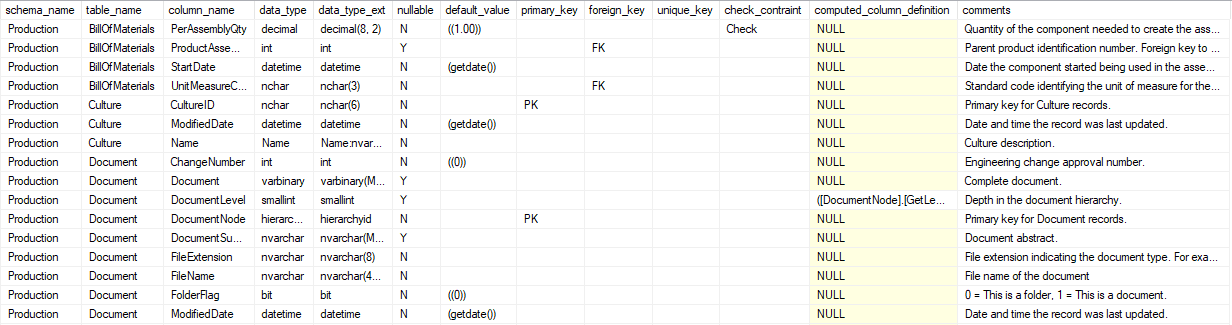## 4. Foreign keys

This query returns list of tables and their foreign keys.

### Query

``````select schema_name(tab.schema_id) as table_schema_name,
tab.name as table_name,
col.name as column_name,
fk.name as constraint_name,
schema_name(tab_prim.schema_id) as primary_table_schema_name,
tab_prim.name as primary_table_name,
col_prim.name as primary_table_column,
schema_name(tab.schema_id) + '.' + tab.name + '.' +
col.name + ' = ' + schema_name(tab_prim.schema_id) + '.' +
tab_prim.name + '.' + col_prim.name as join_condition,
case
when count(*) over (partition by fk.name) > 1 then 'Y'
else 'N'
end as complex_fk,
fkc.constraint_column_id as fk_part
from sys.tables as tab
inner join sys.foreign_keys as fk
on tab.object_id = fk.parent_object_id
inner join sys.foreign_key_columns as fkc
on fk.object_id = fkc.constraint_object_id
inner join sys.columns as col
on fkc.parent_object_id = col.object_id
and fkc.parent_column_id = col.column_id
inner join sys.columns as col_prim
on fkc.referenced_object_id = col_prim.object_id
and fkc.referenced_column_id = col_prim.column_id
inner join sys.tables as tab_prim
on fk.referenced_object_id = tab_prim.object_id
order by table_schema_name,
table_name,
primary_table_name,
fk_part;
``````

### Rows

One row represents one pair of columns in foreign key.

### Columns

Column Meaning
TABLE_SCHEMA_NAME Foreign table schema name.
TABLE_NAME Foreign table name.
COLUMN_NAME Foreign table column name.
CONSTRAINT_NAME Constraint name.
PRIMARY_TABLE_SCHEMA_NAME Primary table schema name.
PRIMARY_TABLE_NAME Primary table name.
PRIMARY_TABLE_COLUMN Primary table column name.
JOIN_CONDITION Join condition containing foreign and primary key tables and columns.
COMPLEX_FK Complex foreign key flag. "Y" when foreign key is complex, otherwise "N".
FK_PART Represents part number of foreign key.

### Sample results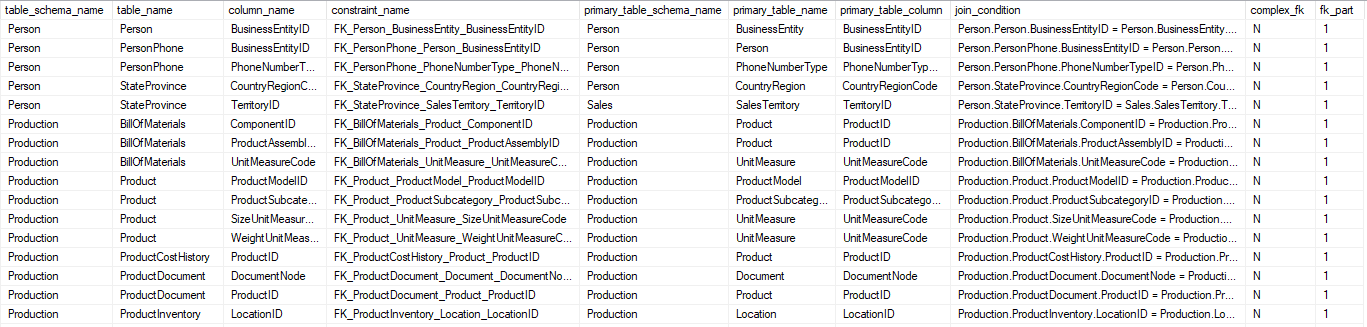## 5. Views columns

This query returns list of views with their columns.

### Query

``````select schema_name(v.schema_id) as schema_name,
v.name as view_name,
col.name as column_name,
t.name as data_type,
t.name +
case when t.is_user_defined = 0 then
isnull('(' +
case when t.name in ('binary', 'char', 'nchar',
'varchar', 'nvarchar', 'varbinary') then
case col.max_length
when -1 then 'MAX'
else
case
when t.name in ('nchar',
'nvarchar') then
cast(col.max_length/2
as varchar(4))
else cast(col.max_length
as varchar(4))
end
end
when t.name in ('datetime2',
'datetimeoffset', 'time') then
cast(col.scale as varchar(4))
when t.name in ('decimal', 'numeric') then
cast(col.precision as varchar(4)) + ', ' +
cast(col.scale as varchar(4))
end + ')', '')
else ':' +
(select c_t.name +
isnull('(' +
case when c_t.name in ('binary', 'char',
'nchar', 'varchar', 'nvarchar',
'varbinary') then
case c.max_length
when -1 then 'MAX'
else case when t.name in
('nchar',
'nvarchar')
then cast(c.max_length/2
as varchar(4))
else cast(c.max_length
as varchar(4))
end
end
when c_t.name in ('datetime2',
'datetimeoffset', 'time') then
cast(c.scale as varchar(4))
when c_t.name in ('decimal', 'numeric') then
cast(c.precision as varchar(4)) +
', ' + cast(c.scale as varchar(4))
end + ')', '')
from sys.columns as c
inner join sys.types as c_t
on c.system_type_id = c_t.user_type_id
where c.object_id = col.object_id
and c.column_id = col.column_id
and c.user_type_id = col.user_type_id
)
end as data_type_ext,
case when col.is_nullable = 0 then 'N' else 'Y' end as nullable,
from sys.views as v
join sys.columns as col
on v.object_id = col.object_id
left join sys.types as t
on col.user_type_id = t.user_type_id
left join sys.extended_properties as ep
on v.object_id = ep.major_id
and col.column_id = ep.minor_id
and ep.name = 'MS_Description'
and ep.class_desc = 'OBJECT_OR_COLUMN'
order by schema_name,
view_name,
column_name;
``````

### Rows

One row represents one view column.

### Columns

Column Meaning
SCHEMA_NAME Schema name.
VIEW_NAME View name.
COLUMN_NAME View column name.
DATA_TYPE Data type. For instance, varchar or decimal.
DATA_TYPE_EXT Data type with information about scale/precision or string length. For instance, varchar(100) or decimal(8, 2).
NULLABLE Nullable flag. "Y" if column is nullable, "N" if column is not nullable.

### Sample results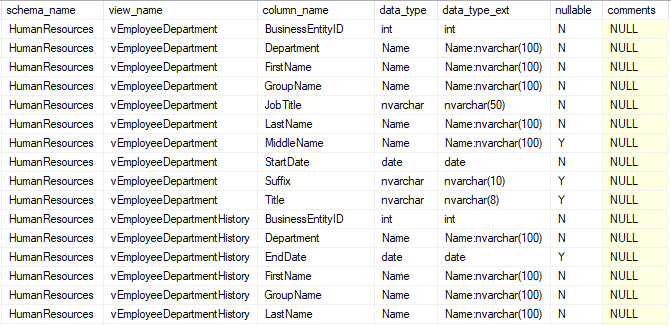## 6. Tables by number of columns

This query returns list of tables sorted by the number of columns they contain.

### Query

``````select schema_name(tab.schema_id) as schema_name,
tab.name as table_name,
count(*) as columns
from sys.tables as tab
inner join sys.columns as col
on tab.object_id = col.object_id
group by schema_name(tab.schema_id),
tab.name
order by count(*) desc;
``````

### Rows

One row represents one user table.

### Columns

Column Meaning
SCHEMA_NAME Schema name.
TABLE_NAME Table name.
COLUMNS Number of columns table contains.

### Sample results